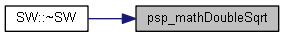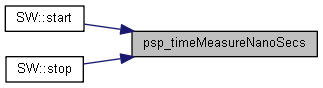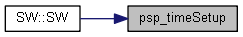55_perf_cpp/sw.h File Reference
`#include "rdmretcodeapi.h"`
`#include <string>`
`#include <iostream>`
`#include <iomanip>`
Include dependency graph for 55_perf_cpp/sw.h:class SW

## Functions

RDM_EXPORT RDM_RETCODE psp_timeSetup (void)
RDM_EXPORT uint64_t psp_timeMeasureNanoSecs (void)
RDM_EXPORT double psp_mathDoubleSqrt (double dv)

## psp_mathDoubleSqrt()

 RDM_EXPORT double psp_mathDoubleSqrt ( double dv )

Referenced by SW::~SW().

Here is the caller graph for this function:## psp_timeMeasureNanoSecs()

 RDM_EXPORT uint64_t psp_timeMeasureNanoSecs ( void )

Referenced by SW::start(), and SW::stop().

Here is the caller graph for this function:## psp_timeSetup()

 RDM_EXPORT RDM_RETCODE psp_timeSetup ( void )

Referenced by SW::SW().

Here is the caller graph for this function: